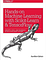# Build A Simple Machine Learning Python Program

Program Your Own Machine Learning Model

We are going to create a simple machine learning program (the model) using the programming language called Python and a supervised learning algorithm called Linear Regression from the sklearn library (A.K.A scikit-learn). We will create a training data set of pseudo-random integers as input by using the Python library Random, and create our own function for the training data set output using those random integer values as input. Then we will train our model on the newly created data set and finally test our model with one example to see how well our model performed to predict/ give the expected output.

# Step 0: Install Python Version 3

https://realpython.com/installing-python/

# Step 1: Install Scikit-Learn

First we need to install our dependency, the Python library scikit-learn (sklearn) which is a free software machine learning library for the Python Programming Language. The random library is part of the python standard libraries so we don’t need to install it, it’s already available for us !

`pip install scikit-learn`

# Step 2: Create Your Training Data Set

Usually if you have a data set, you would want to split it into a training set, validation set, and testing set. Since this is a simple example we are creating our own training set to train our linear regression model with.The training set will contain the input and expected output values of the data set that I want to train the model on. The input will be random integer values created by the library Random we will use the method randint(a,b) , which returns a random integer N such that `a <= N <= b`. The output will be determined by a function that we will create.

Recall a function usually called ‘f’ takes in some input/parameter value usually called ‘x’ and produces some output value, such that f(x) = g. The function that I will create will take in multiple parameters so not just ‘x’ but ‘x’, ‘y’, and ‘z’ instead such that we get the following function.

f(x,y,z) = 10x + 2y + 3z = g

Example of how this function works, if we had values x=1, y=2, z=20 then y would equal 74 like the following:

f(1,2,20) = 10(1) + 2(2) + 3(20) = 74

Input = Features
Output = Targets

# Code To Generate Training Set:

`# Import the librariesfrom randomfrom sklearn.linear_model import LinearRegression# Create an empty list for the feature data set 'X' and the target data set 'y'feature_set = []target_set= []# get the number of rows wanted for the data setnumber_of_rows = 200# limit the possible values in the data setrandom_number_limit = 2000#Create the training data set#Create and append a randomly generated data set to the input and outputfor i in range(0,number_of_rows):  x = random.randint(0, random_number_limit)  y = random.randint(0, random_number_limit)  z = random.randint(0, random_number_limit)#Create a linear function for the target data set 'y'function = (10*x) + (2*y) + (3*z)feature_set.append([x,y,z])target_set.append(function)`

# Step 3: Train Our Linear Regression Model

Next we will use the method LinearRegression() from the Python library scikit-learn to train and create our model. This model will try to “model” the function that we created for the training dataset.

Note: When we ‘fit’ a function that is just another word for training.

`model = LinearRegression() #Create a linear regression object/modelmodel.fit(feature_set, target_set) `

# Step 4: Test The Linear Regression Model

Now let’s see if our LinearRegression function can approximate the function that we created and give us an accurate prediction (the correct answer).

Test Set= [[8, 10, 0]]

The outcome should be 8*10 + 10*2 + 0*3 = 100. Let’s see what we got…

`test_set = [[8,10,0]] prediction = model.predict(test_set)print('Prediction:'+str(prediction)+'\t'+ 'Coefficient:'+str(model.coef_))`

Prediction : [ 100.] Coefficients : [ 8. 10. 0.]

The model used the training data, to compute the coefficients also known as weights. It then used the weights with the feature / input data to compute the correct output. When giving the model test data, it successfully managed to get the correct answer!

If you are also interested in reading up a little bit more on machine learning to immediately get started with problems and examples then I strongly recommend you check out Hands-On Machine Learning with Scikit-Learn and TensorFlow: Concepts, Tools, and Techniques to Build Intelligent Systems.Hands-On Machine Learning with Scikit-Learn and TensorFlow: Concepts, Tools, and Techniques to Build Intelligent Systems

Written by